# 20 To Positive And Negative Number Line PrintableUpdated on Feb 11, 2021By Printablee Team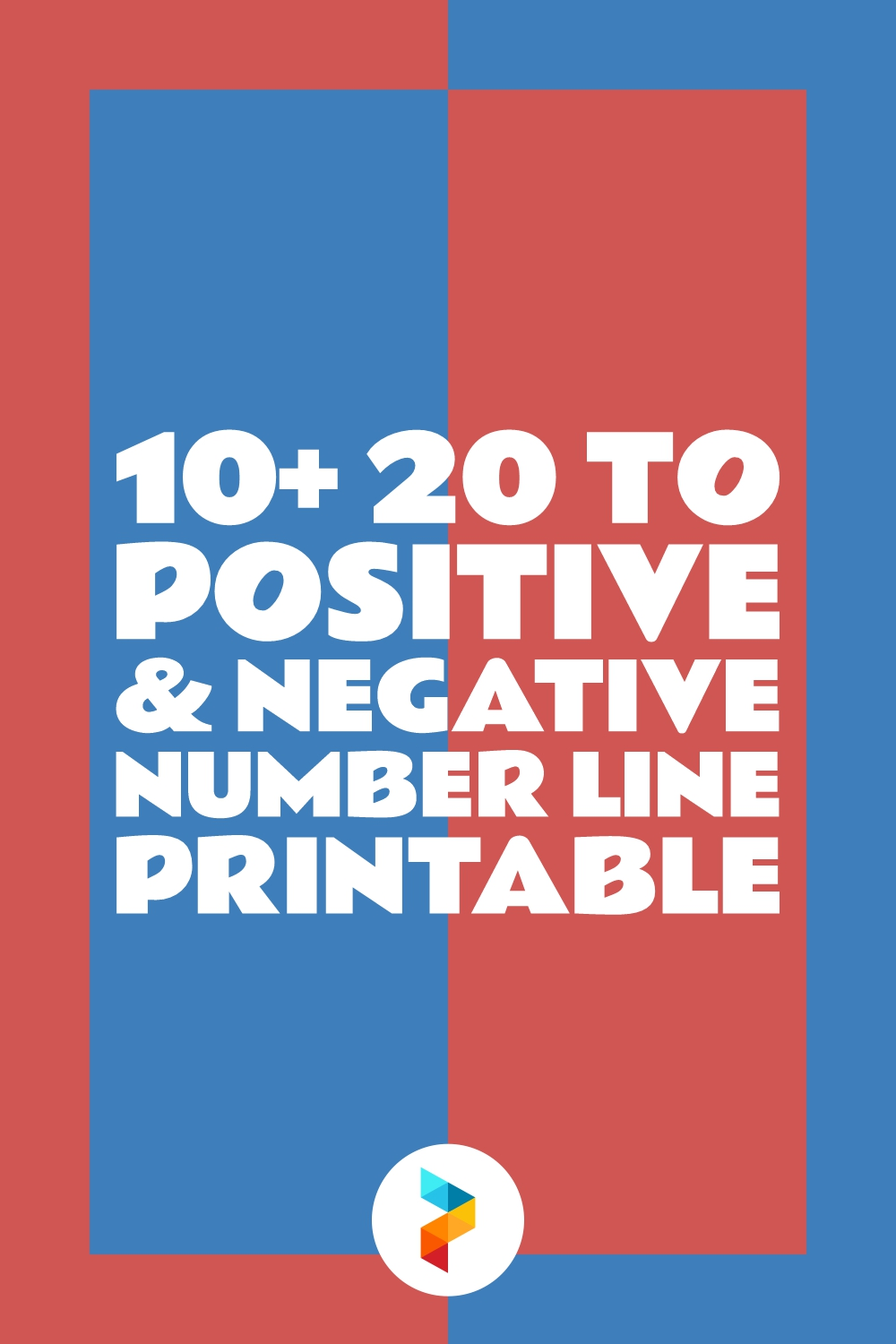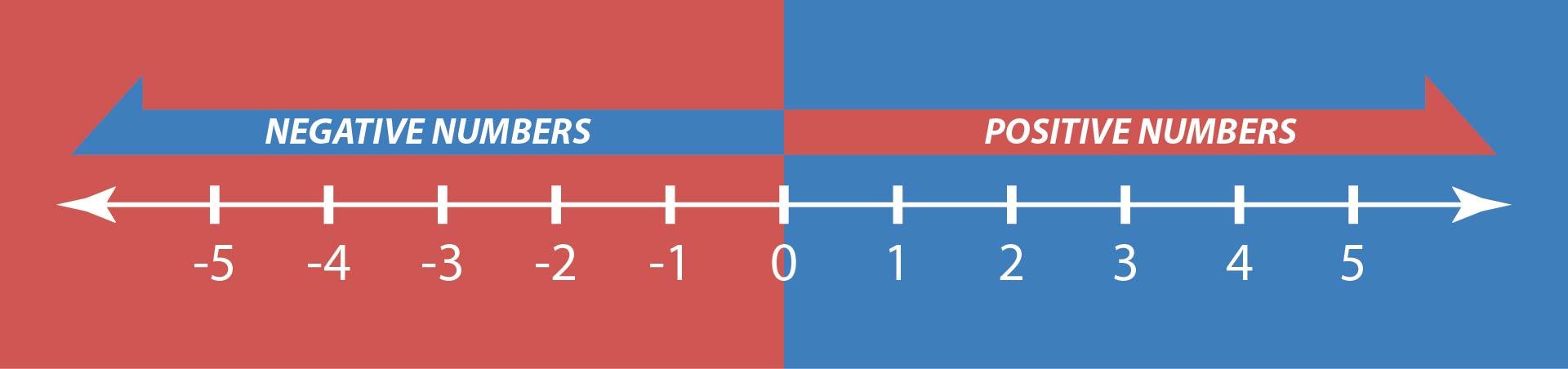## What are Positive and Negative Numbers?

Numbers that are greater than zero are called positive numbers and numbers that are less than zero are called negative numbers. Therefore, to distinguish between them, the sign (+) is used for positive numbers and (-) for negative numbers. For positive numbers, usually the sign is not written. Meanwhile, the sign (-) must be given to negative numbers.

The easiest way to visualize positive and negative numbers is to use a number line. The number line is a horizontal line consisting of negative numbers and positive numbers. Zero is written in the middle of the number line. With a number line, the calculation of positive and negative numbers will also be easier.

The calculation of positive and negative numbers includes various arithmetic operations such as addition, subtraction, multiplication, and division. So, to calculate positive and negative numbers, you need to understand the basic rules of these positive and negative numbers. Therefore, let's discuss positive and negative numbers: the basic rules and why it is useful for real life.

## What are the Basic Rules of Positive and Negative Numbers?

Understanding the basic rules of positive and negative numbers is important because these two types of numbers are often used in everyday life. In general, the basic rules for positive and negative numbers are as follows.

• The value of a positive number is greater than zero. This number has a positive sign. However, it is usually written without a sign. An example is +4 written as 4.
• Meanwhile, the value of a negative number is less than zero. The sign for negative numbers is (-) and the sign must be written in front of the number. Examples are -2 and -3.
• Zero is not a positive number or a negative number.
• The sum of positive numbers and negative numbers with the same value is zero. An example is 2-2 = 0.
• Operations on negative numbers are easier to do with parentheses. Examples are 2-(-3) or 3+(-5).
• A number line can make calculating positive and negative numbers easier.
• There are specific rules for adding, multiplying, subtracting, and dividing positive and negative numbers.

Here are the rules for arithmetic operations on positive and negative numbers.

• Adding positive or negative numbers with the same sign results in a number with the same sign. Examples are (-2) + (-3) = -5 or 3 + 2 = 5.
• Subtraction of positive and negative numbers is done by subtracting a large number from a small number. Then, the sign is obtained from a large number. An example is (-5) + 3 = -2.
• Multiplication between two positive numbers gives a positive value and multiplication between two negative numbers gives a negative value.
• If a positive number is multiplied by a negative number, the result is negative.
• Multiplying any positive or negative number by zero will result in a zero answer.

## Why are Positive and Negative Numbers Useful for Real Life?

Positive and negative numbers are widely used in life such as determining temperature, counting money, using elevators, determining sea levels, and playing quizzes or games.

Some jobs are also related to positive and negative numbers. Examples are financial and banking institutions. Several fields of science also require knowledge regarding positive and negative numbers such as knowing signs of underweight in patients.

Not only that, positive and negative numbers are also used in sports. That number is used to calculate the points in cricket, golf, baseball, and rugby matches.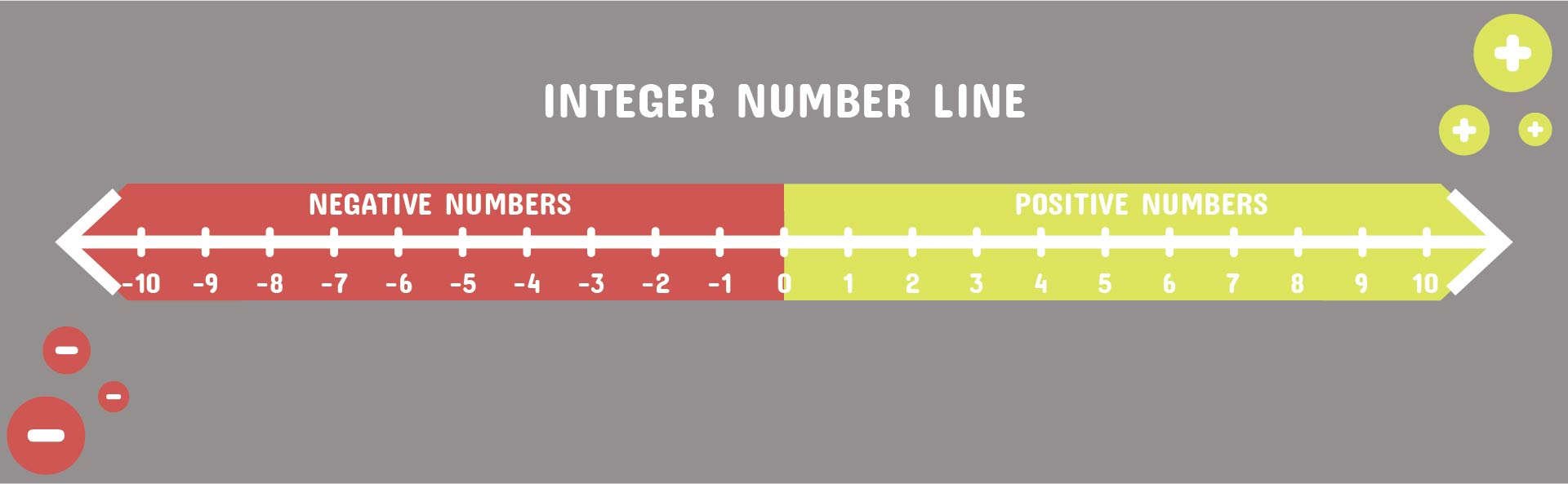We also have more printable number you may like:
Printable Number Lines To 20
Number Lines 0 20 Printable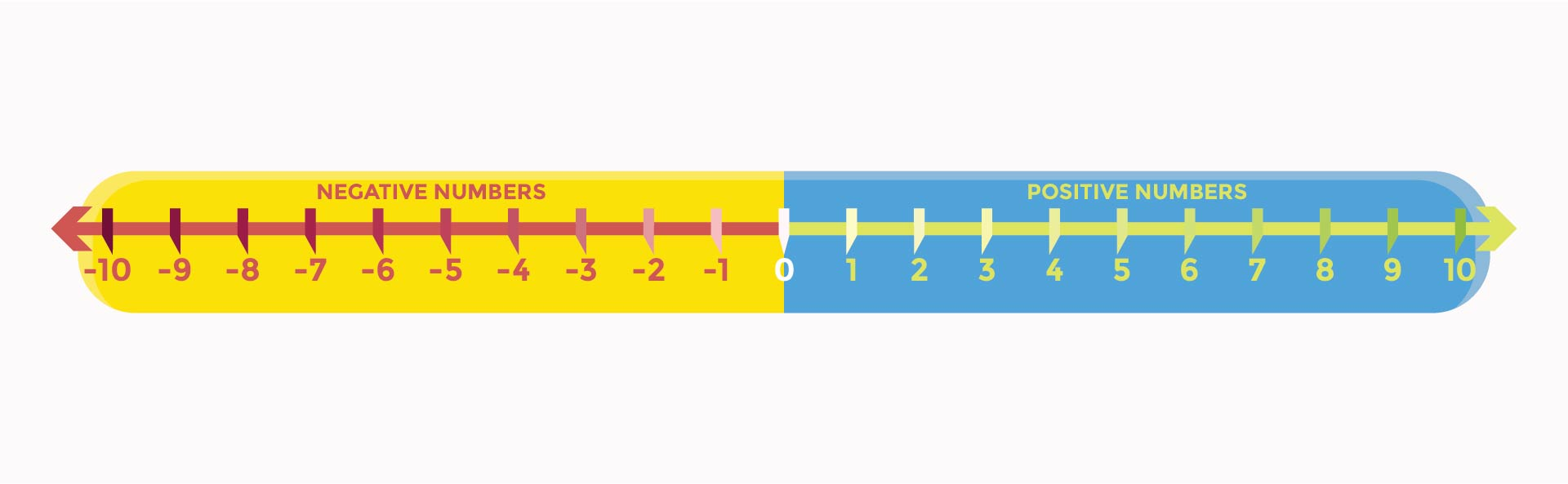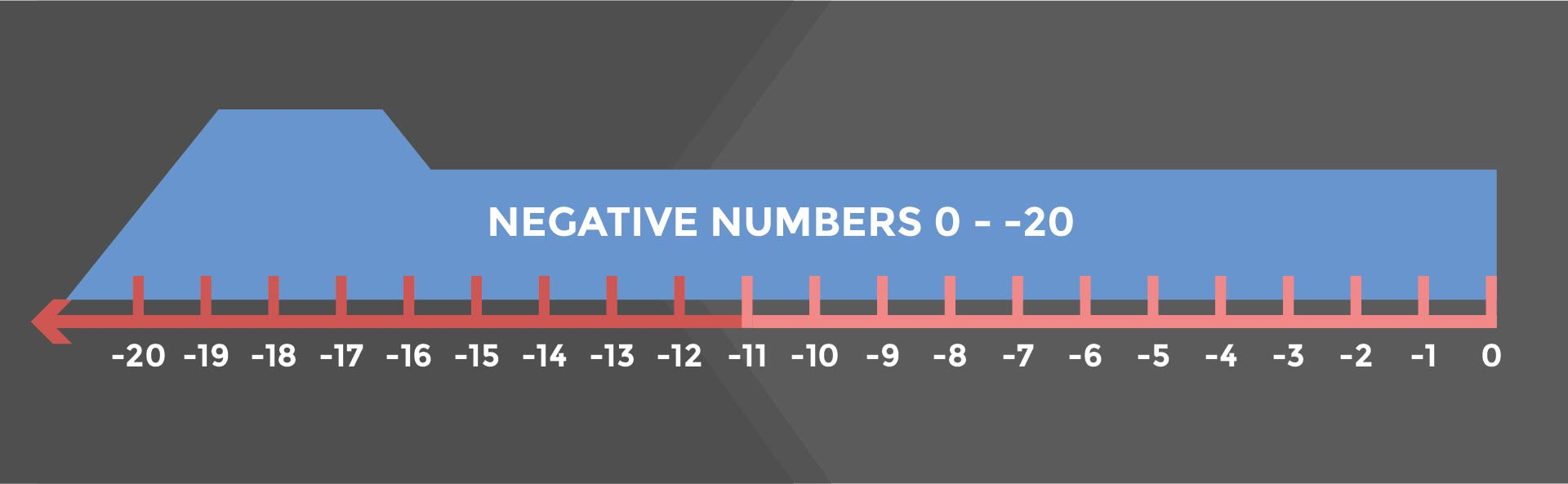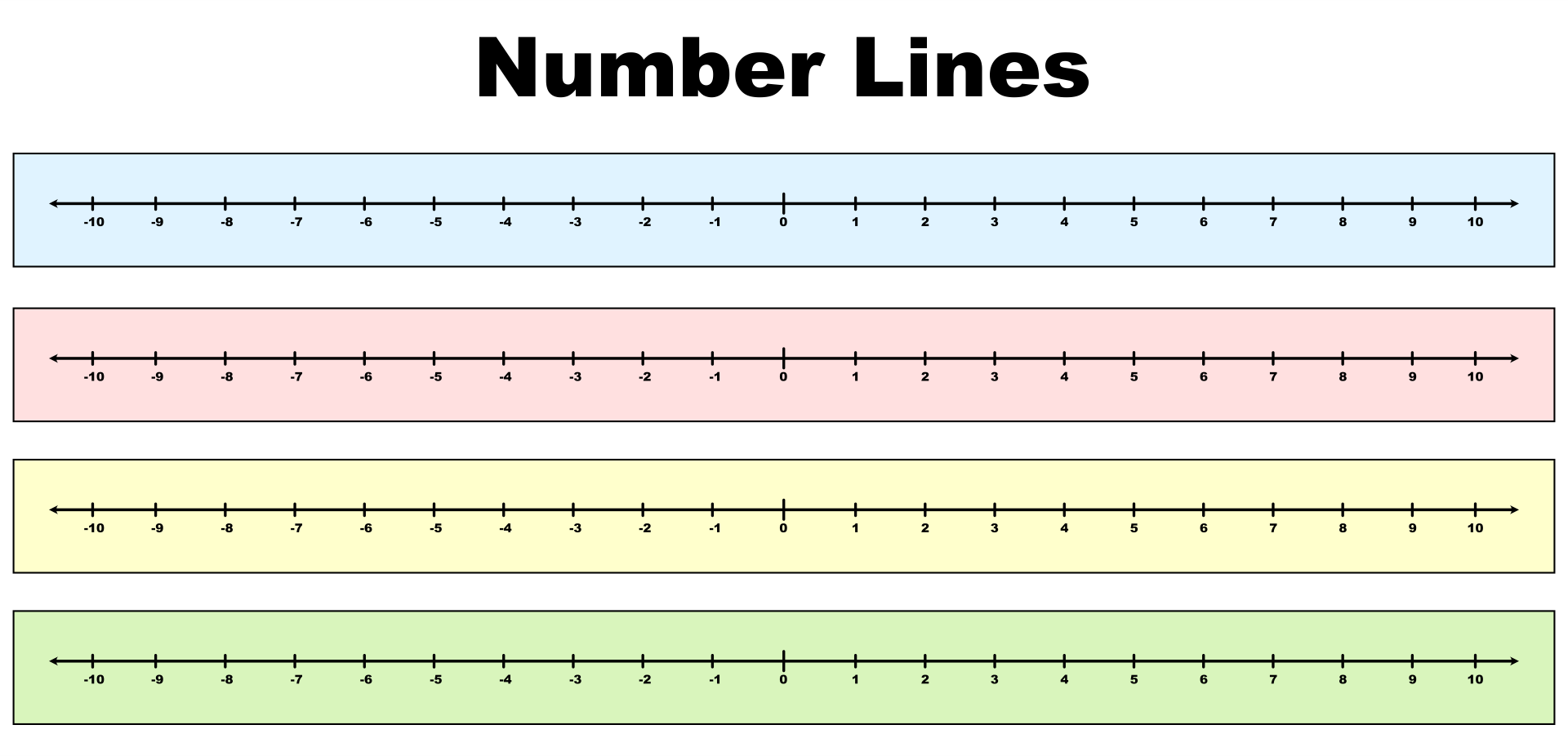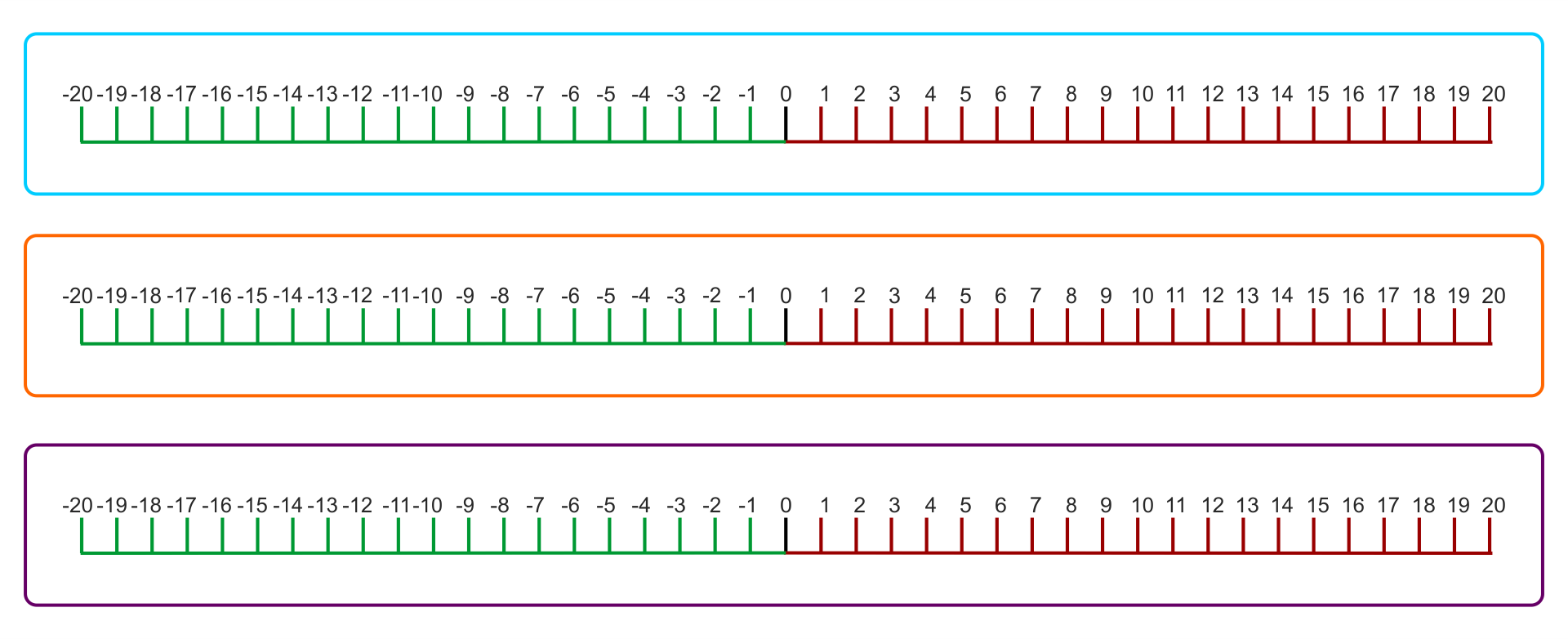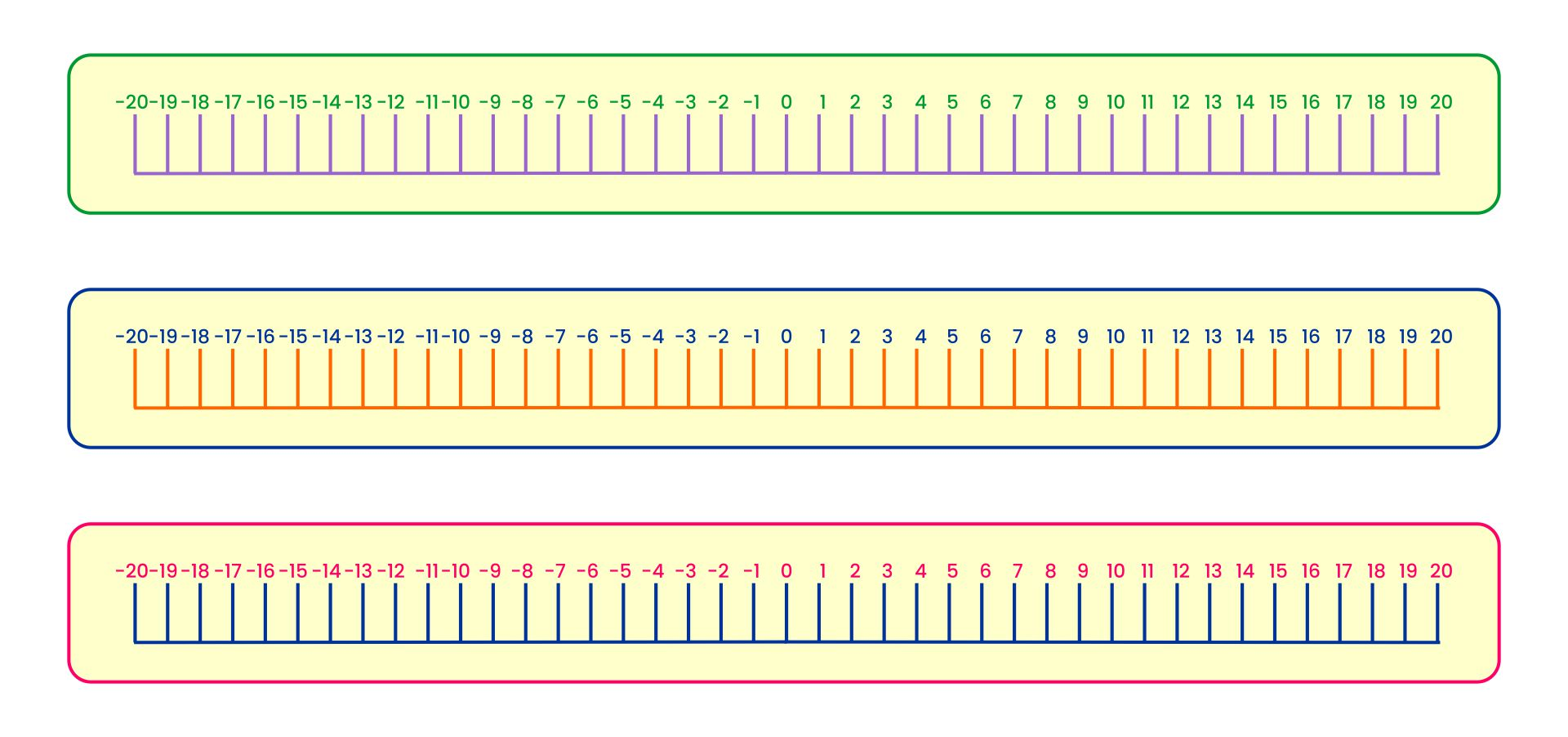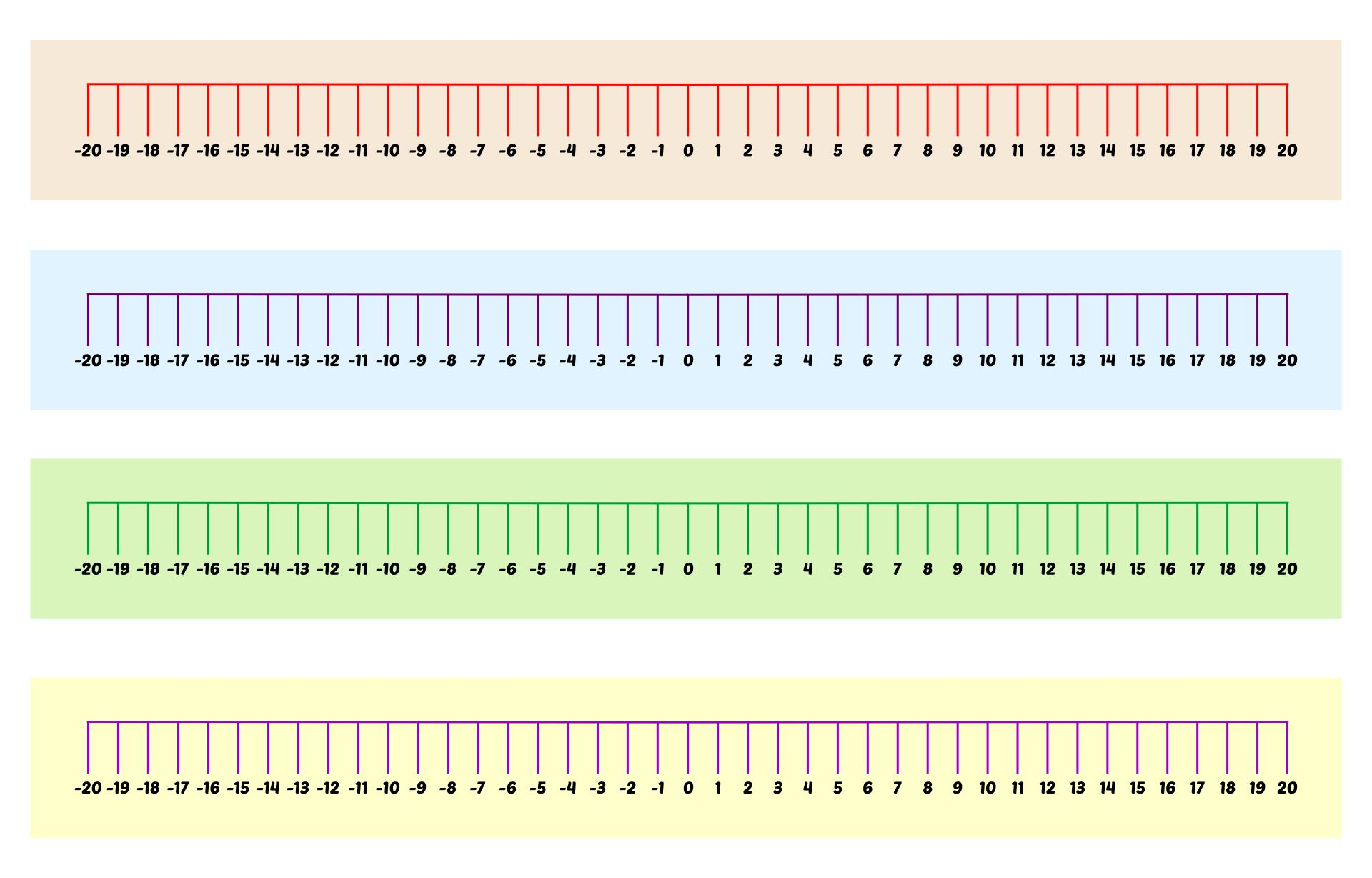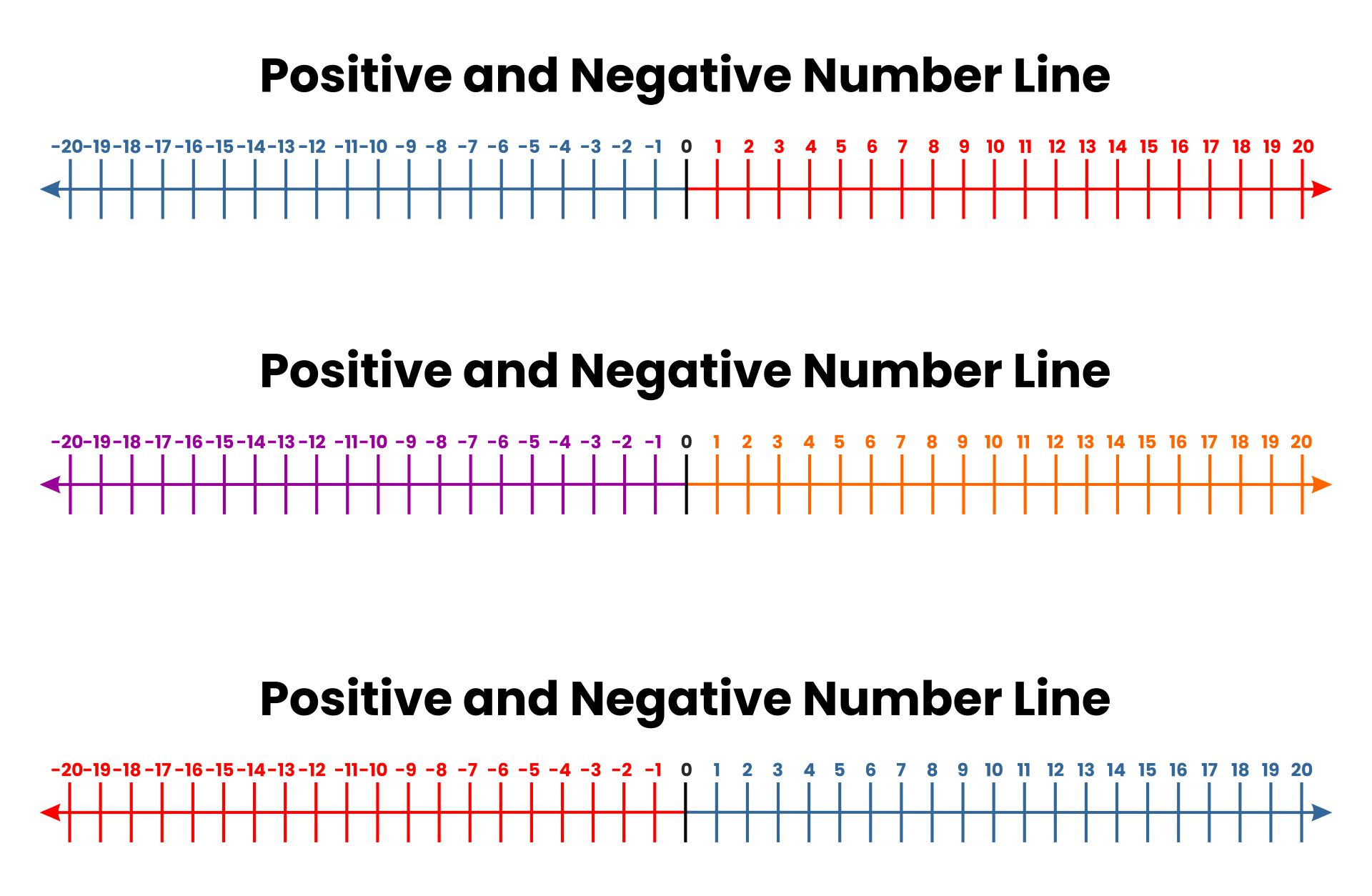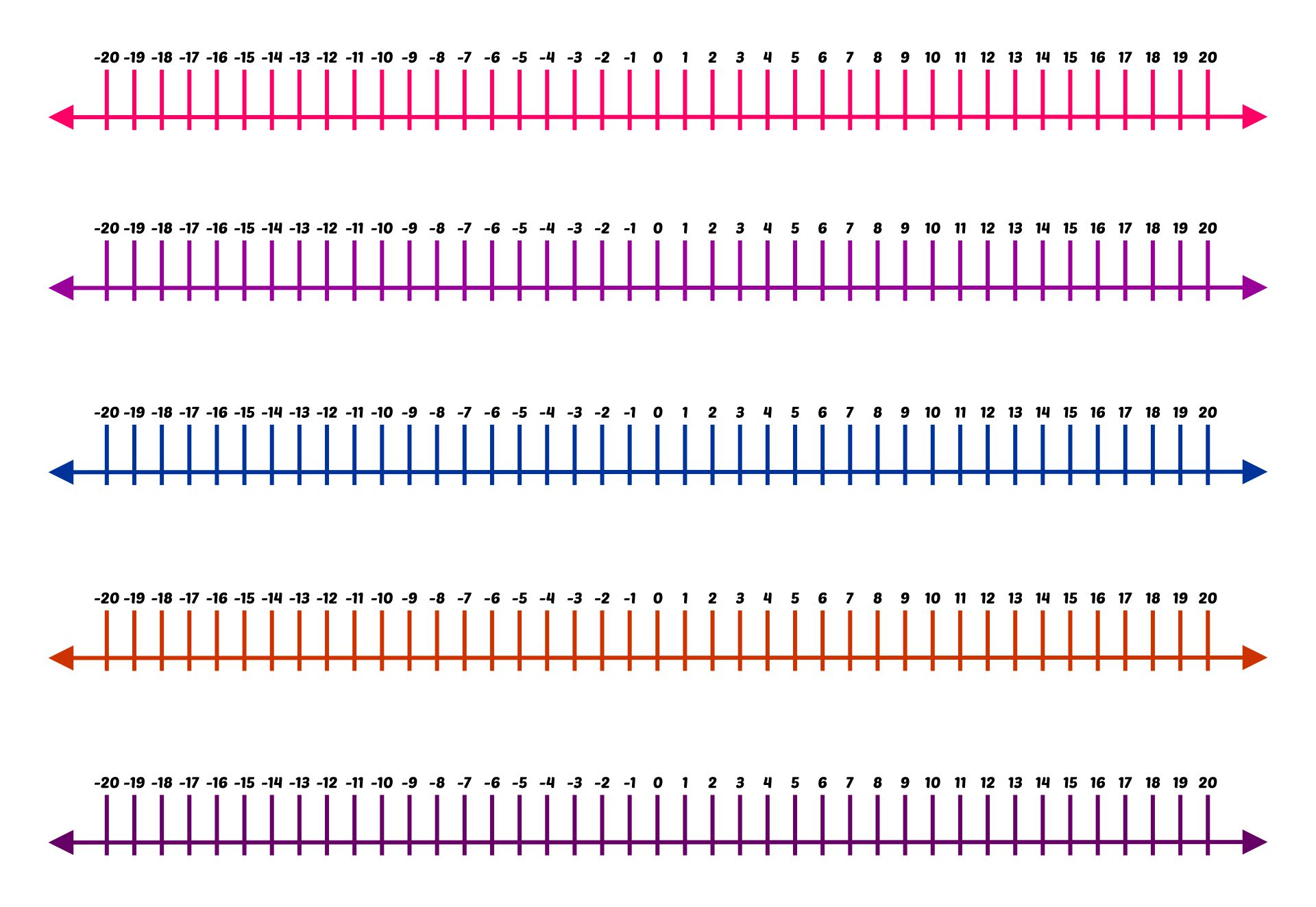## What are the Positive and Negative Numbers Activities for Kids?

Because positive and negative numbers are very important for everyday life, you need to teach these types of numbers to children. So, here are some positive and negative number activities that you can do with your children.

• For children aged 5-8 years, you can teach them about positive and negative numbers through numbers and board games. One type of board game is Escape the Pyramid. There are positive and negative numbers on the board game. When they could get closer to the exit, they would earn positive points. They will get negative points if they have to go down.

• Make some cards containing math challenges about positive and negative numbers. So, there is a problem with the positive and negative numbers on each of these cards. Then, ask the children to complete the challenge on all of these cards.

• Children can also learn about positive and negative numbers through number puzzle worksheets. Just download the number puzzle worksheet and print it. There are several questions in the puzzles. The children must complete the calculation of the positive and negative numbers. Then, they must write their answers in the box provided. So, it’s like a crossword game.

• Children are usually happy when playing a quiz. Well, you can make a numbers quiz about positive and negative numbers. So, all the questions in the quiz are about arithmetic operations on positive and negative numbers.

• Give the children several scenarios of positive and negative numbers. Then, ask them to solve the scenario using the basic rules of positive and negative numbers. So, you can use numbers in a context worksheet to do this one activity. Just download the worksheets and print the worksheets.

• Children can also learn positive and negative numbers by placing positive or negative numbers in order. Just like other activities, you can use worksheets that can be downloaded and printed.